# Switching Boost Regulator: Design Basics and Efficiency

Published  August 3, 2018   0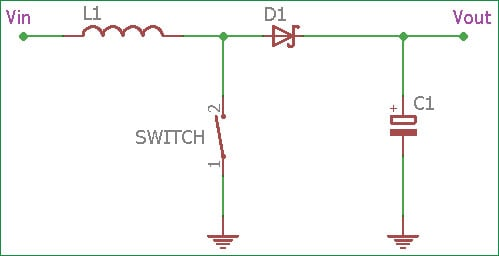In electronics, a regulator is a device or mechanism which can regulate power output constantly. There are different kinds of regulators available in the power supply domain. But mainly, in the case of DC to DC conversion, there are two types of regulators available: Linear or Switching.

A linear regulator regulates the output using a resistive voltage drop, and due to this Linear regulators provide lower efficiency and lose power in the form of heat.

On the other side Switching regulator use inductor, Diode and a power switch to transfer energy from its source to the output.

There are three types of switching regulators available.

1.Step-up  converter (Boost Regulator)

2.Step-Down converter (Buck regulator)

3.Inverter (Flyback)

In this tutorial we describe the Switching Boost Regulator circuit. We already described the Boost Regulator Design in the previous tutorial. Here we will discuss different aspects of Boost converter and how to improve its efficiency.

### Design Basics of Boost Converter Circuit

In many cases, we need to convert lower voltage to the higher voltage depending on the requirements. Boost regulator boosts the voltage from lower potential to higher potential.In the above image, a simple Boost regulator circuit is shown where an Inductor, diode, Capacitor and a switch are used.

The purpose of the inductor is to limit the current slew rate which is flowing through the power switch. It will limit the excess high-peak current that is unavoidable by the switch resistance individually.

Also, the inductor stores energy, the energy measured in Joules E = (L * I2 / 2)

We will understand how the inductors transfer energy in the upcoming images and graphs.

In case of switching boost regulators, there are two Phases, One is Inductor Charge phase or the Switch on phase (Switch is closed actually) and the other one is Discharge phase or the switch off phase (Switch is open).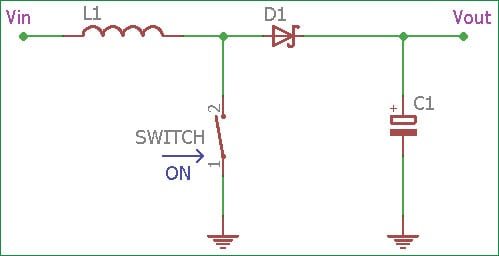If we assume that the switch has been in open position for a long time, the voltage drop across the diode is negative and the voltage across the capacitor is equal to the input voltage. In this situation, if the switch gets close the Vin is frightened across the inductor. The Diode prevents the capacitor discharge through the switch to the ground.

The current through the inductor rises at linearly with time. The linear current rising rate is proportional to the input voltage divided by the inductance di / dt = Voltage across Inductor / Inductance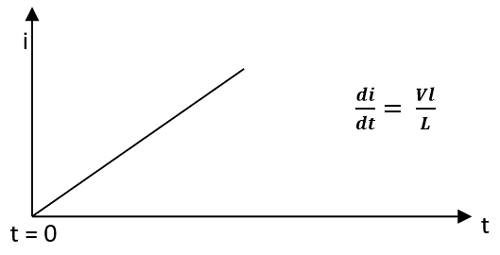In the upper graph, showing the Charging phase of the inductor. The x-axis denotes t (time) and the Y-axis denotes I (current through the inductor). The Current is increasing linearly with time when the switch is closed or ON.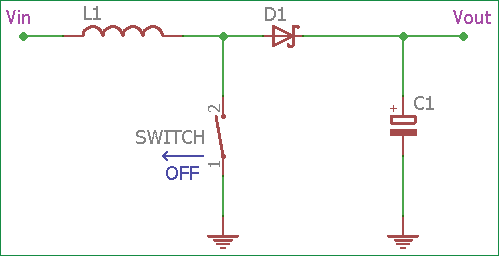Now, when the switch again gets off or became open, the inductor current flow through the diode and charge the output capacitor. When the output voltage rises, the current slope through the inductor reverses. The Output voltage rises until Voltage through the inductor = L * (di / dt) is reached.

The Inductor current drop rate with time is directly proportional to the inductor voltage. Higher the inductor voltage, faster the current drop through the inductor.In the above graph, the inductor current drops with time when the switch becomes off.

When the switching regulator is in steady-state operating condition, Inductor’s average voltage is Zero during the entire switching cycle. For this condition, the average current through the inductor is also in steady-state.

If we assume that the inductor charge time is Ton and the circuit has an input voltage, then there will be a specific Toff or discharge time for an output voltage.

As the average inductor voltage is equal to zero in steady state we can construct boost circuit using following terms

```Vin X Ton = Toff x VL
VL = Vin x (Ton / Toff)```

As the output voltage is equal to the Input voltage and average inductor voltage (Vout = Vin + VL)

We can say that,

```Vout = Vin + Vin x (Ton / Toff)
Vout = Vin x ( 1 +  Ton / Toff)```

We can also calculate the Vout using duty cycle.

```Duty Cycle (D) = Ton / (Ton + Toff)
```

For the boost switching regulator the Vout will be Vin / ( 1 – D )

### PWM and Duty Cycle for Boost Converter Circuit

If we control the duty cycle, we can control the steady-state output of the boost converter. So, for the duty cycle variation, we use a control circuit across the switch.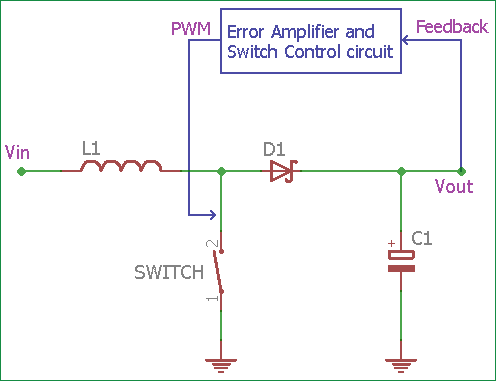So, for a complete basic boost regulator circuit, we need an additional circuitry which will vary the duty cycle and thus the amount of time the inductor receives energy from the source.

In the above image, an Error amplifier can be seen which sense the output voltage across the load using a feedback path and control the switch. Most common control technic includes PWM or Pulse Width Modulation technic which is used to control the duty cycle of the circuitry.

The control circuit controls the amount of time the switch remains open or close, depending on the current drawn by the load. This circuit also uses for continuous operation in the steady state. It will take a sample of the output voltage and to subtract it from a reference voltage and create a small error signal, then this error signal will be compared to an oscillator ramp signal and from the comparator output a PWM signal will operate or control the switch circuit.

When the output voltage changes, the error voltage also affected by it. Due to error voltage change, the comparator controls the PWM output. The PWM also changed to a position when the output voltage creates zero error voltage and by doing this, the closed control loop system executes the work.

Fortunately, Most modern Switching boost regulators have this thing inbuilt inside the IC package. Thus simple circuitry design is achieved using the modern switching regulators.

The reference feedback voltage is done using a resistor divider network. This is the additional circuitry, which is needed along with inductor, diodes, and capacitors.

### Improve efficiency of Boost Converter Circuit

Now, If we investigate about the efficiency, It is how much power we provide inside the circuitry and how much we get at the output.

`(Pout / Pin) * 100%`

As energy cannot be created nor destroyed, it can only be converted, most electrical energies loose unused powers converted to heat. Also, there is no ideal situation in the practical field, efficiency is a larger factor for selecting Voltage regulators.

One of the main power-loss factors for a switching regulator is the diode. The forward voltage drop times current (Vf x i) is the unused wattage which converted to heat and reduces the efficiency of the switching regulator circuit. Also, It is the additional cost to the circuitry for thermal/heat management technics using a heatsink, or Fans to cool down the circuitry from dissipated heat. Not only the forward voltage drop, Reverse recovery for silicon diodes also produce unnecessary power loss and reduction of the overall efficiency.

One of the best way to avoid a standard recovery diode is to use Schottky diodes in place of diodes that have a low forward voltage drop and better reverse recovery. When maximum efficiency is needed, the diode can be replaced using MOSFETs. In modern technology, there are plenty of choices available in Switching boost regulator section, which provide more than 90% efficiency easily.

Also, there is a feature “Skip Mode” which is being used in many modern devices which allows the regulator to skip switching cycles when there is no need of switching at very light loads. It is a great way to improve efficiency in light load condition. In skip mode, Switching cycle is initiated only when the output voltage drops below a regulating threshold.

Despite having Higher efficiency, Stationary design technic, smaller component, switching regulators are noisy than a linear regulator.  Still, they are widely popular.

### Example Design for Boost Converter

We previously created a boost regulator circuit using MC34063 where the 5V output is generated from the 3.7V input voltage. MC34063 is the switching regulator which was used in boost regulator configuration. We used an Inductor, a Schottky diode, and capacitors.In the above image, Cout is the output capacitor and we also used an inductor and Schottky diode which are the basic components for a switching regulator. There is also a Feedback network used. R1 and R2 resistors create a voltage divider circuit which is needed for the comparator's PWM and error amplification stage. The reference voltage of the comparator is 1.25V.

If we see the project in detail, we can see that 70-75% efficiency is achieved by this MC34063 switching boost regulator circuit. Further efficiency can be improved using proper PCB technic and obtaining thermal management procedures.